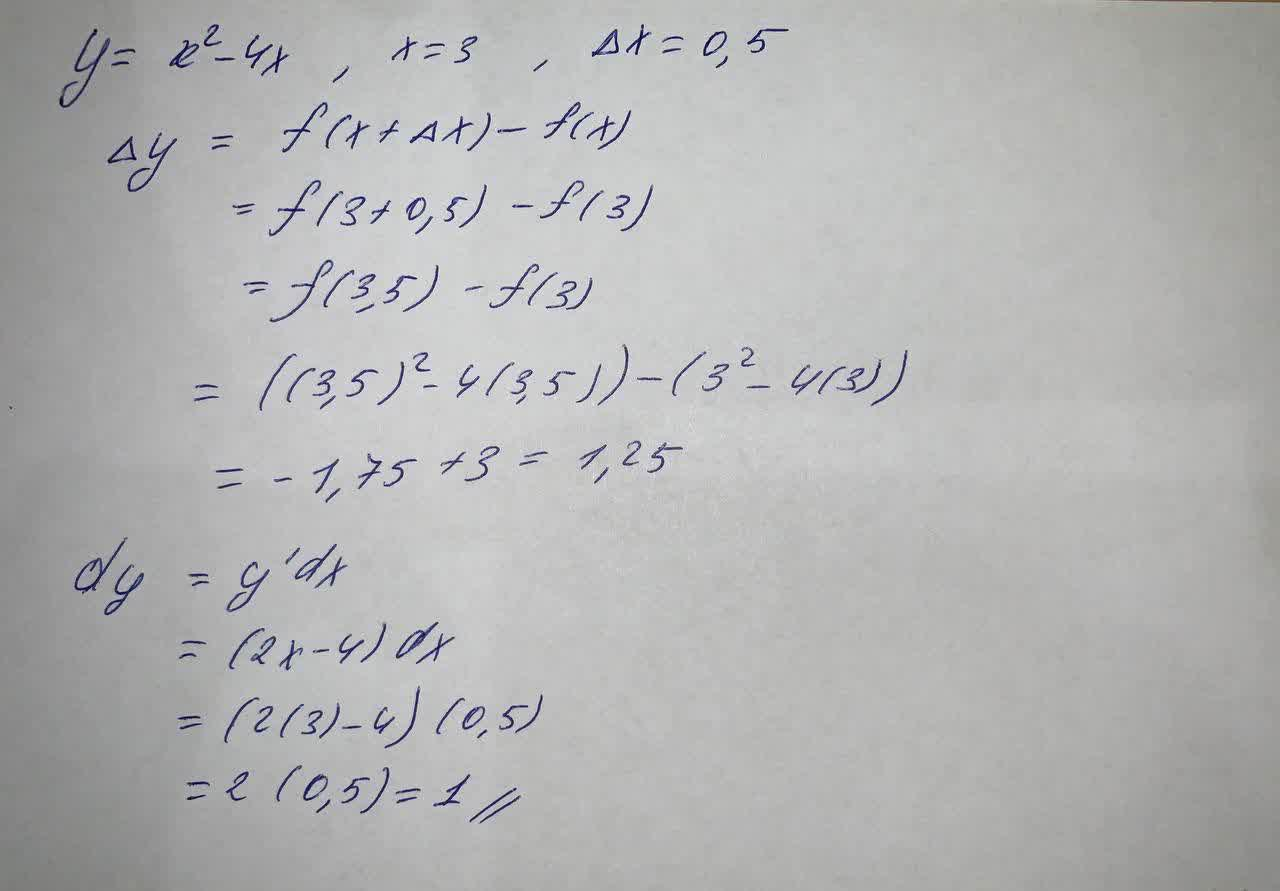Compute \triangle y and dy for the given values of x and dx=\triangle x y=x^2-4x, x=3 , \triangle x =0,5 \triangle y=??? dy=?aflacatn 2021-05-17 Answered
Compute $$\triangle y$$ and dy for the given values of x and $$dx=\triangle x$$
$$y=x^2-4x, x=3 , \triangle x =0,5$$
$$\triangle y=???$$
dy=?

• Questions are typically answered in as fast as 30 minutes

Solve your problem for the price of one coffee

• Math expert for every subject
• Pay only if we can solve itirwchh